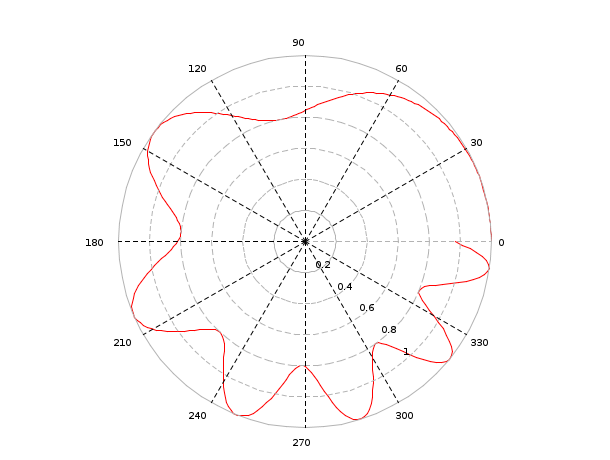Change language to:
Français - 日本語 - Português - Русский

See the recommended documentation of this function

Scilab help >> Graphics > 2d_plot > polarplot

# polarplot

Plot polar coordinates

### Calling Sequence

```polarplot(theta,rho,[style,strf,leg,rect])
polarplot(theta,rho,<opt_args>)```

### Arguments

rho

theta

a vector with same size than rho, the angle values.

<opt_args>

a sequence of statements `key1=value1, key2=value2`, ... where keys may be `style`,`leg`,`rect`,`strf` or `frameflag`

style

is a real row vector of size nc. The style to use for curve `i` is defined by `style(i)`. The default style is `1:nc` (1 for the first curve, 2 for the second, etc.).

-

if `style(i)` is negative, the curve is plotted using the mark with id `abs(style(i))+1`; use `xset()` to see the mark ids.

-

if `style(i)` is strictly positive, a plain line with color id `style(i)` or a dashed line with dash id `style(i)` is used; use `xset()` to see the color ids.

-

When only one curve is drawn, `style` can be the row vector of size 2 `[sty,pos]` where `sty` is used to specify the style and `pos` is an integer ranging from 1 to 6 which specifies a position to use for the caption. This can be useful when a user wants to draw multiple curves on a plot by calling the function `plot2d` several times and wants to give a caption for each curve.

strf

is a string of length 3 `"xy0"`.

default

The default is `"030"`.

x

controls the display of captions,

x=0

no captions.

x=1

captions are displayed. They are given by the optional argument `leg`.

y

controls the computation of the frame. same as frameflag

y=0

the current boundaries (set by a previous call to another high level plotting function) are used. Useful when superposing multiple plots.

y=1

the optional argument `rect` is used to specify the boundaries of the plot.

y=2

the boundaries of the plot are computed using min and max values of `x` and `y`.

y=3

like `y=1` but produces isoview scaling.

y=4

like `y=2` but produces isoview scaling.

y=5

like `y=1` but `plot2d` can change the boundaries of the plot and the ticks of the axes to produce pretty graduations. When the zoom button is activated, this mode is used.

y=6

like `y=2` but `plot2d` can change the boundaries of the plot and the ticks of the axes to produce pretty graduations. When the zoom button is activated, this mode is used.

y=7

like `y=5` but the scale of the new plot is merged with the current scale.

y=8

like `y=6` but the scale of the new plot is merged with the current scale.

leg

a string. It is used when the first character x of argument `strf` is 1. `leg` has the form `"leg1@leg2@...."` where `leg1`, `leg2`, etc. are respectively the captions of the first curve, of the second curve, etc. The default is `""`.

rect

This argument is used when the second character y of argument `strf` is 1, 3 or 5. It is a row vector of size 4 and gives the dimension of the frame: `rect=[xmin,ymin,xmax,ymax]`.

### Description

polarplot creates a polar coordinate plot of the angle theta versus the radius rho. theta is the angle from the x-axis to the radius vector specified in radians; rho is the length of the radius vector specified in dataspace units. Note that negative rho values cause the corresponding curve points to be reflected across the origin.

### Example 1

```t= 0:.01:2*%pi;
clf();polarplot(sin(7*t),cos(8*t))```### Example 2

```t= 0:.01:2*%pi;

clf();polarplot([sin(7*t') sin(6*t')],[cos(8*t') cos(8*t')],[1,2])```### Example 3

```t = 0:0.01:2*%pi;
polarplot(t, -1 + sin(t));```### Example 4

```clf()
theta=[0:0.02:2*%pi]';
rho=1+0.2*cos(theta.^2)
polarplot(theta,rho,style=5)
a=gca()
a.isoview='on'
a.data_bounds=[-1.2,-1.2;1.2,01.2]```Report an issue << plot2d4 2d_plot 3d_plot >>